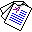# Abductive Equivalence in First-order Logic

## Katsumi Inoue and Chiaki Sakama

Logic Journal of the IGPL, 14(2):333-346, Oxford University Press, 2006.

## Abstract

In Artificial Intelligence, abduction is often formalized in first-order logic. In this article, we focus on the problem of identifying equivalence of two abductive theories represented in first-order logic. To this end, two definitions of equivalence are given for abduction. Explainable equivalence requires that two abductive theories have the same explainability for any observation. On the other hand, explanatory equivalence guarantees that any observation has exactly the same explanations in each abductive theory. Explanatory equivalence is a stronger notion than explainable equivalence. In first-order logic, explainable equivalence can be veri.ed by the notion of extensional equivalence in default theories, while explanatory equivalence reduces to logical equivalence between background theories. We also show the complexity results for abductive equivalence.Full Paper available here